## 【LeetCode周赛】2022上半年题目精选集——思维题

article2023/10/2 20:32:40/

### 文章目录

• 2211. 统计道路上的碰撞次数（栈 || 脑筋急转弯）
• 解法1：自己想的——使用栈
• 解法2——思维：去掉左右两边往左右开的车
• 代码写法1——找左右端点
• 代码写法2——正则表达式去除+流处理api
• 补充：replaceAll() 和 正则表达式
• 补充：(int) s.chars().filter(c -> c == 'S').count(); 解释
• 2227. 加密解密字符串 （逆向思维）
• 思路
• 代码1
• 代码2
• 2242. 节点序列的最大得分⭐⭐⭐⭐⭐ （有技巧的枚举）
• 思路——有技巧的枚举（枚举中间的边）
• 代码
• 2306. 公司命名⭐⭐⭐⭐⭐（分类讨论）
• 2317. 操作后的最大异或和（位运算）
• 思路
• 代码
• 相关链接

## 2211. 统计道路上的碰撞次数（栈 || 脑筋急转弯）

2211. 统计道路上的碰撞次数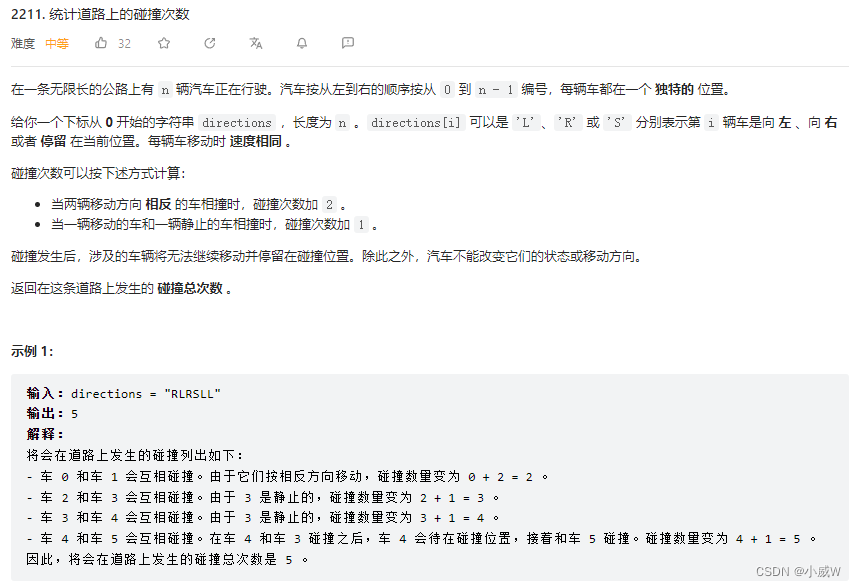1 <= directions.length <= 10^5
directions[i] 的值为 'L'、'R' 或 'S'

### 解法1：自己想的——使用栈

class Solution {
public int countCollisions(String directions) {
Deque<Character> stk = new ArrayDeque();
int ans = 0;
for (char ch: directions.toCharArray()) {
if (ch == 'L') {
if (!stk.isEmpty()) {
stk.push('S');
++ans;
}
} else stk.push(ch);
}
boolean f = false;
while (!stk.isEmpty()) {
char ch = stk.pop();
if (ch == 'R' && f) ++ans;
if (ch != 'R') f = true;
}
return ans;
}
}


### 解法2——思维：去掉左右两边往左右开的车

#### 代码写法1——找左右端点

class Solution {
public int countCollisions(String directions) {
int n = directions.length(), l = 0, r = n - 1, ans = 0;
while (l < n && directions.charAt(l) == 'L') ++l;
while (r >= 0 && directions.charAt(r) == 'R') --r;
for (int i = l; i <= r; ++i) {
if (directions.charAt(i) != 'S') ++ans;
}
return ans;
}
}


#### 代码写法2——正则表达式去除+流处理api

class Solution {
public int countCollisions(String s) {
// 前缀向左的车不会发生碰撞
s = s.replaceAll("^L+", "");
// 后缀向右的车不会发生碰撞
s = new StringBuilder(s).reverse().toString().replaceAll("^R+", "");
// 剩下非停止的车必然会碰撞
return s.length() - (int) s.chars().filter(c -> c == 'S').count();
}
}

##### 补充：replaceAll() 和 正则表达式

Java 中的 String 类的 replaceAll() 方法是用来使用给定的 replacement 字符串替换此字符串匹配给定的正则表达式的每个子字符串。

public String replaceAll(String regex, String replacement)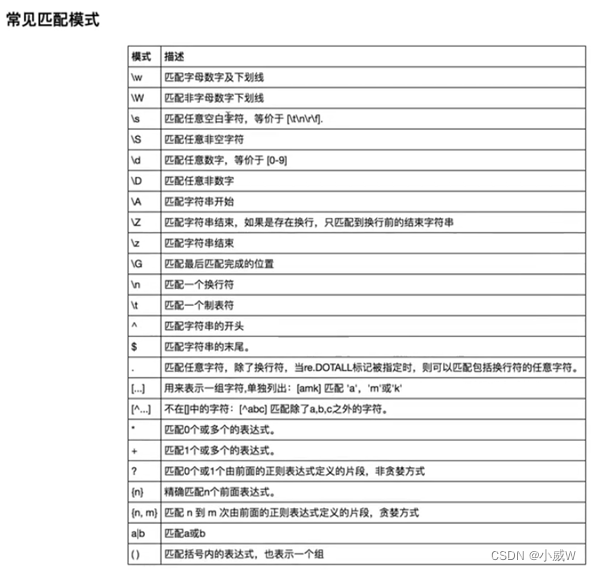##### 补充：(int) s.chars().filter(c -> c == ‘S’).count(); 解释

return s.length() - (int) s.chars().filter(c -> c == 'S').count();


## 2227. 加密解密字符串 （逆向思维）

2227. 加密解密字符串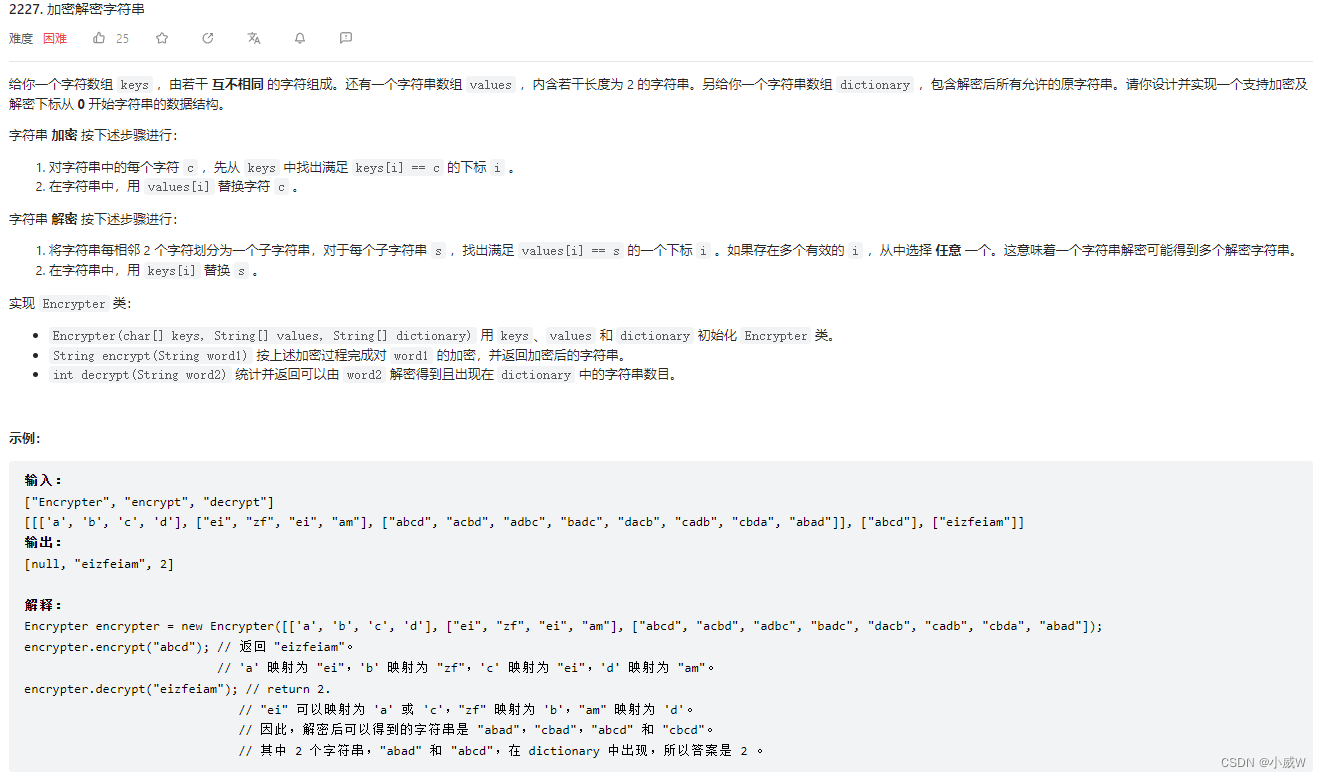1 <= keys.length == values.length <= 26
values[i].length == 2
1 <= dictionary.length <= 100
1 <= dictionary[i].length <= 100
所有 keys[i] 和 dictionary[i] 互不相同
1 <= word1.length <= 2000
1 <= word2.length <= 200
所有 word1[i] 都出现在 keys 中
word2.length 是偶数
keys、values[i]、dictionary[i]、word1 和 word2 只含小写英文字母
至多调用 encrypt 和 decrypt 总计 200 次

### 思路

class Encrypter {

public Encrypter(char[] keys, String[] values, String[] dictionary) {

}

public String encrypt(String word1) {

}

public int decrypt(String word2) {

}
}


• 构造方法用来定义一些会被使用到的数据结构。
• 加密方法按照题目要求进行模拟即可。
• 为了计算解密后出现在 dictionary 中字符串的数量，我们可以提前预处理出所有 dictionary 加密后的字符串并计数保存，这样在解密方法中直接查表就好了。

### 代码1

class Encrypter {
char[] keys;
String[] values, dictionary;
Map<Character, Integer> m = new HashMap();
Map<String, Integer> ans = new HashMap();

public Encrypter(char[] keys, String[] values, String[] dictionary) {
this.keys = keys;
this.values = values;
this.dictionary = dictionary;
// 存储key中字符和对应的下标
for (int i = 0; i < keys.length; ++i) m.put(keys[i], i);
// 提前计算加密结果
for (String d: dictionary) {
String dd = encrypt(d);
// 这里使用 dd + d.length() 作为键是为了确保我们找的加密前字符串的长度是解密前字符串长度的1/2，直接使用dd的话会被最后一个样例卡住
// 即a对应bb,bbbb应该被解密成aa，但abb这个字符串加密之后也会成为bbbb影响答案计算
ans.merge(dd + d.length(), 1, Integer::sum);
}
}

public String encrypt(String word1) {
StringBuilder ans = new StringBuilder();
for (char ch: word1.toCharArray()) {
if (m.containsKey(ch)) ans.append(values[m.get(ch)]);
else ans.append(ch);
}
return ans.toString();
}

public int decrypt(String word2) {
// 直接查表即可
return ans.getOrDefault(word2 + (word2.length() / 2), 0);
}
}


### 代码2

// 这里使用 dd + d.length() 作为键是为了确保我们找的加密前字符串的长度是解密前字符串长度的1/2，直接使用dd的话会被最后一个样例卡住
// 即a对应bb,bbbb应该被解密成aa，但abb这个字符串加密之后也会成为bbbb影响答案计算


class Encrypter {
Map<String, Integer> cnt = new HashMap();
String[] map = new String;

public Encrypter(char[] keys, String[] values, String[] dictionary) {
// 记录字符c对应keys中下标i所在的values[i]
for (int i = 0; i < keys.length; ++i) {
map[keys[i] - 'a'] = values[i];
}
// 预处理dictionary加密
for (String s: dictionary) {
String e = encrypt(s);
cnt.merge(e, 1, Integer::sum);
}
}

public String encrypt(String word1) {
StringBuilder res = new StringBuilder();
for (char ch: word1.toCharArray()) {
String s = map[ch - 'a'];
if (s == null) return "";
res.append(s);
}
return res.toString();
}

public int decrypt(String word2) {
return cnt.getOrDefault(word2, 0);
}
}


## 2242. 节点序列的最大得分⭐⭐⭐⭐⭐ （有技巧的枚举）

2242. 节点序列的最大得分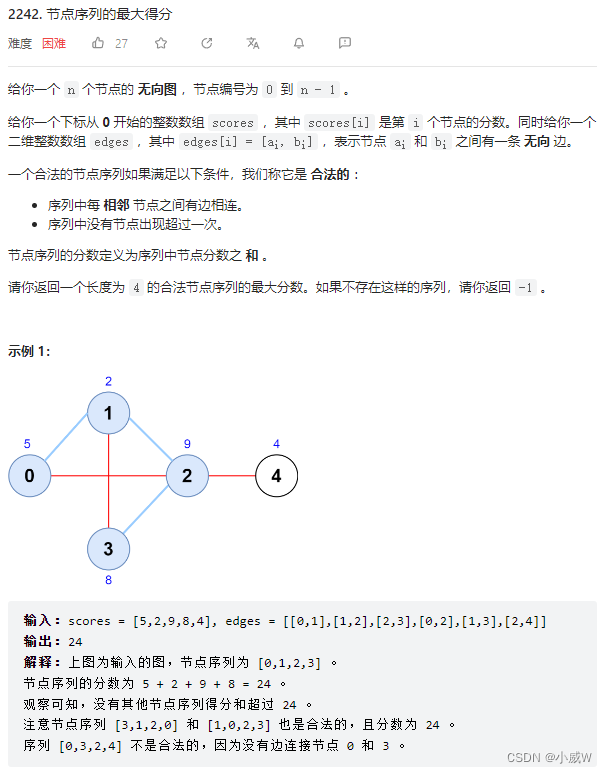### 思路——有技巧的枚举（枚举中间的边）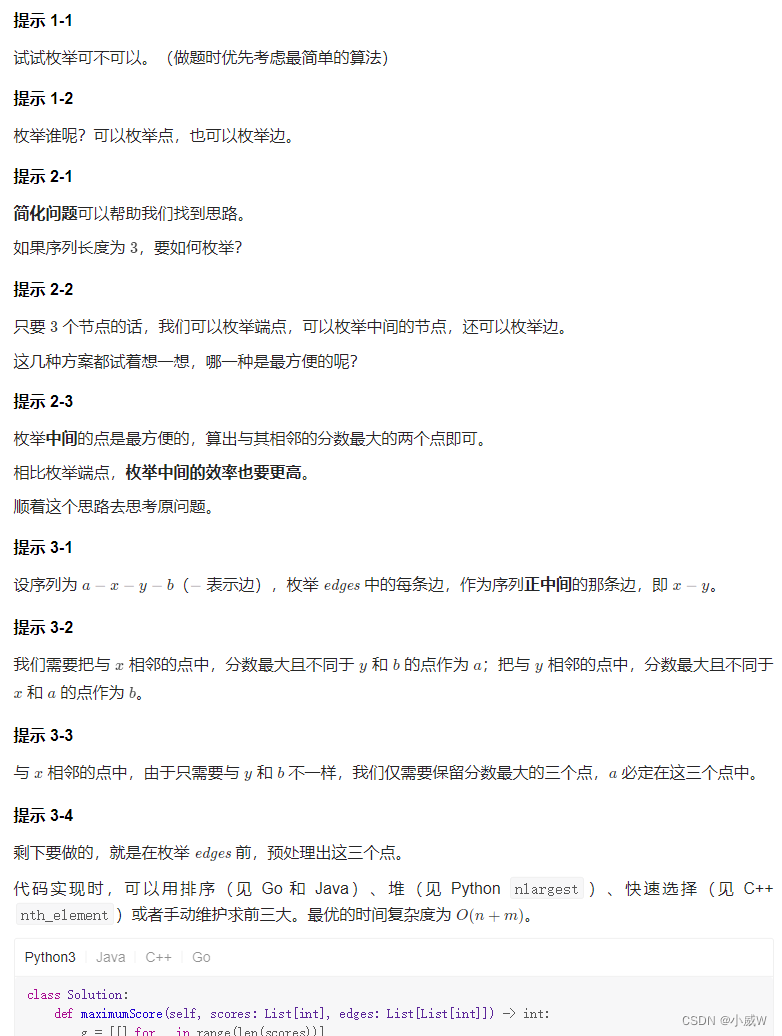Q：为什么枚举中间的边效率最好？
A：因为枚举一条边的同时就会确定两个点，这时只需要再对这两个点找到相邻最大的点就可以了。

### 代码

class Solution {
public int maximumScore(int[] scores, int[][] edges) {
int n = scores.length;
List<int[]>[] g = new ArrayList[n];
Arrays.setAll(g, e -> new ArrayList());
for (int[] edge: edges) {
int x = edge, y = edge;
}
for (int i = 0; i < n; ++i) {
// 如果和i相连的点的数量>3，就可以删掉只剩3个最大的
// 这样删可以确保和它组成一个序列的另外3个值都不会被删掉
// 即对于序列a-x-y-b,枚举到x的时候要保证a,y,b都不会被删掉
// 删去其它的边是为了后面遍历的时候快一些
if (g[i].size() > 3) {
g[i].sort((a, b) -> (b - a));     // 按照score从大到小排序
g[i] = new ArrayList<>(g[i].subList(0, 3));
}
}

int ans = -1;
// 枚举每个边作为中间的边
for (int[] edge: edges) {
int x = edge, y = edge;
for (int[] p: g[x]) {
int a = p;       // x旁边的节点号a
for (int[] q: g[y]) {
int b = q;   // y旁边的节点号b
if (a != y && b != x && a != b) {
ans = Math.max(ans, p + q + scores[x] + scores[y]);
}
}
}
}
return ans;
}
}


## 2306. 公司命名⭐⭐⭐⭐⭐（分类讨论）

2306. 公司命名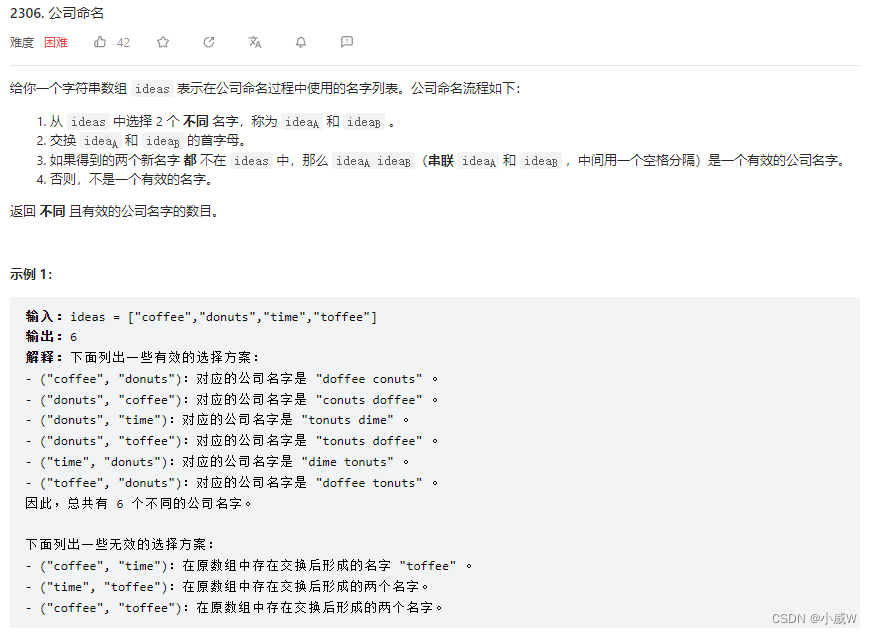2 <= ideas.length <= 5 * 10^4
1 <= ideas[i].length <= 10
ideas[i] 由小写英文字母组成
ideas 中的所有字符串 互不相同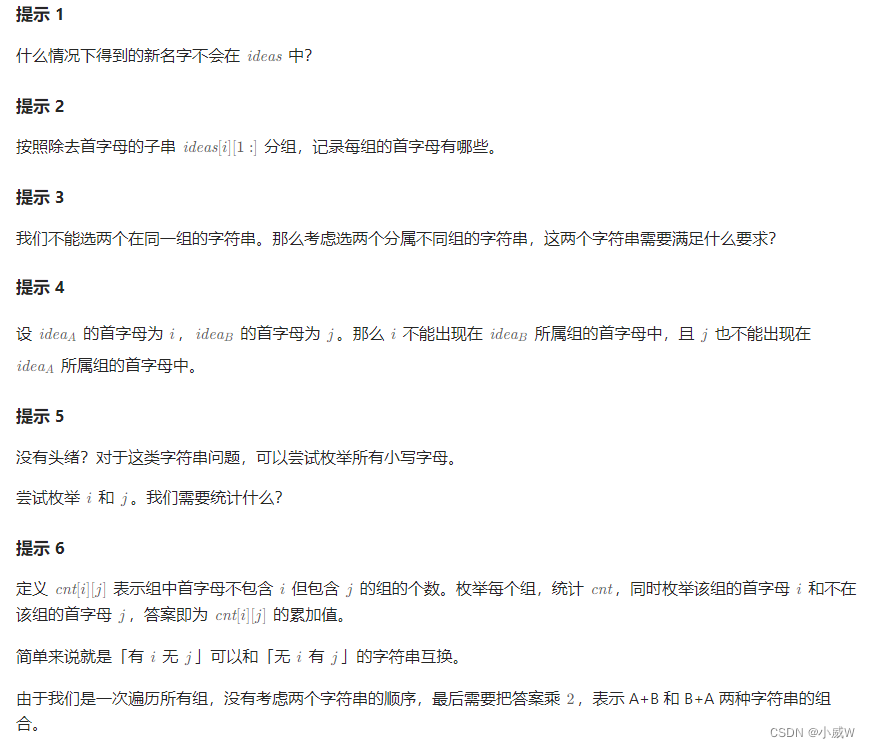class Solution {
public long distinctNames(String[] ideas) {
Map<String, Integer> group = new HashMap();
for (String idea: ideas) {
String t = idea.substring(1);
group.put(t, group.getOrDefault(t, 0) | 1 << (idea.charAt(0) - 'a'));
}

long ans = 0;
int[][] cnt = new int;              // cnt[i][j]表示没i有j的组的个数
// 计算cnt[i][j]
for (int i = 0; i < 26; ++i) {
for (int j = i + 1; j < 26; ++j) {
if ((mask >> i & 1) == 0 && (mask >> j & 1) == 1) ++cnt[i][j];
else if ((mask >> i & 1) == 1 && (mask >> j & 1) == 0) ++cnt[j][i];
}
}
}
// 所有成对的 cnt[i][j]和cnt[j][i] 可以贡献方案数
for (int i = 0; i < 26; ++i) {
for (int j = i + 1; j < 26; ++j) {
ans += cnt[i][j] * cnt[j][i];
}
}
return ans * 2;
}
}


## 2317. 操作后的最大异或和（位运算）

2317. 操作后的最大异或和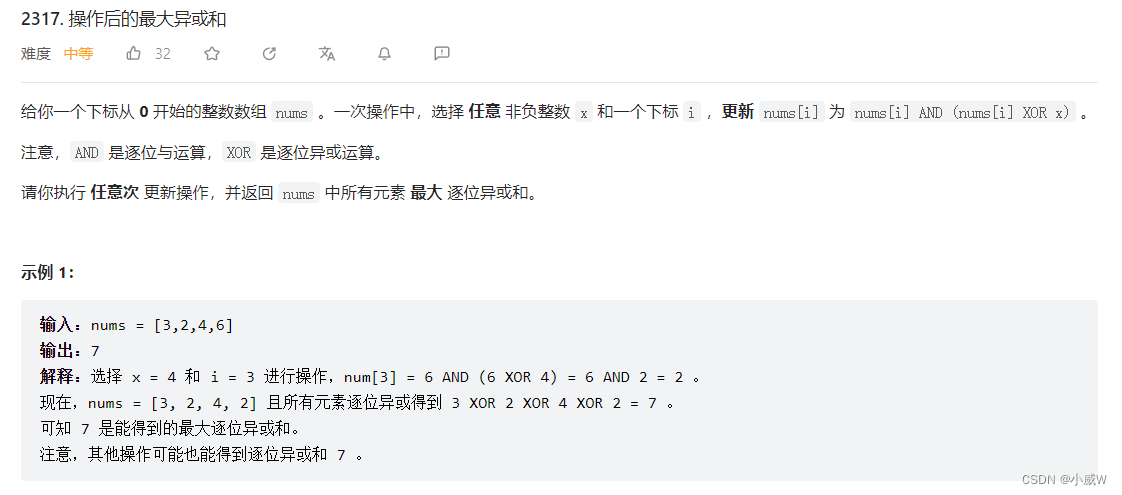### 思路

=》有 1 就保留 1 ，这是 或运算 的性质。

### 代码

class Solution {
public int maximumXOR(int[] nums) {
int ans = 0;
for (int num: nums) ans |= num;
return ans;
}
}


# 相关链接

### python图像处理实战（三）—图像几何变换

&#x1f680;写在前面&#x1f680; &#x1f58a;个人主页&#xff1a;https://blog.csdn.net/m0_52051577?typeblog &#x1f381;欢迎各位大佬支持点赞收藏&#xff0c;三连必回&#xff01;&#xff01; &#x1f508;本人新开系列专栏—python图像处理 ❀愿每一个骤雨初…

### 【MySQL】基本查询之表的增删改查

【MySQL】表的增删改查 一、插入操作----insert1.1 简单插入1.2 插入时是否更新----ON DUPLICATE KEY UPDATE1.3 插入时替换----REPLACE 二、查询----select2.1 简单查询与去重2.2 基本查询----where条件2.2.3 案列演示 2.4 排序----order by 三、修改操作----update四、删除--…

### Spring Boot 集成 Redisson分布式锁

Redisson 是一种基于 Redis 的 Java 驻留集群的分布式对象和服务库&#xff0c;可以为我们提供丰富的分布式锁和线程安全集合的实现。在 Spring Boot 应用程序中使用 Redisson 可以方便地实现分布式应用程序的某些方面&#xff0c;例如分布式锁、分布式集合、分布式事件发布和订…

### Oracle数据库软件安装与卸载

Oracle数据库软件安装与卸载 实验目的及要求 学习Oracle12c数据库服务器软件和客户端软件的安 装与卸载,掌握客户端服务名的设置,建立客户端与服务器的网络连接,熟悉windows操作系统中Oracle相关服务的操作。理解数据库管理的基本架构。 &#xff08;1&#xff09;熟悉Oracle…

### 【macOS 系列】mac设置截屏或其他操作的默认保存位置

1、第一步、在用户/图片文件夹下&#xff0c;新建“截图”文件夹 2、第二步、打开终端&#xff0c;输入defaults write com.apple.screencapture location ~/Pictures/截图/后回车 3、第三步、操作完成后&#xff0c;再次输入killall SystemUIServer后回车 如果你在web前端开发…

### 机器学习一：线性回归

1 知识预警 1.1 线性代数 ( A T ) T A (A^\mathrm{T})^\mathrm{T}A (AT)TA\$ ( A B ) T A T B T (AB)^\mathrm{T}A^\mathrm{T}B^\mathrm{T} (AB)TATBT ( λ A ) T λ A T (\lambda A)^\mathrm{T}\lambda A^\mathrm{T} (λA)TλAT ( A B ) T B T A T (AB)^\mathrm{T}B^…